## Periodic and Symmetric Functions

The unit circle has a circumference of C = 2π r = 2π(1) = 2π. Therefore, if a point P travels around the unit circle for a distance of 2π, it ends up where it started. In other words, for any given value q, if 2π is added or subtracted, the coordinates of point P remain unchanged (Figure  1).Figure  1
Periodic coterminal angles.

It follows that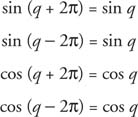If k is an integer,Functions that have this property are called periodic functions. A function f is periodic if there is a positive real number q such that f(x + q) = f(x) for all x in the domain of f. The smallest possible value for q for which this is true is called the period of f.

Example 1: If sin y = y = (3/5)/10, then what is the value of each of the following: sin(y + 8π), sin(y + 6π), (y + 210π)?

All three have the same value of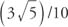because the sine function is periodic and has a period of 2π.

The study of the periodic properties of circular functions leads to solutions of many real‐world problems. These problems include planetary motion, sound waves, electric current generation, earthquake waves, and tide movements.

Example 2: The graph in Figure 2  represents a function f that has a period of 4. What would the graph look like for the interval −10 ⩽ x ⩽ 10?Figure 2
Drawing for Example 2.

This graph covers an interval of 4 units. Because the period is given as 4, this graph represents one complete cycle of the function. Therefore, simply replicate the graph segment to the left and to the right (Figure  3 ).Figure 3
Drawing for Example 2.

The appearance of the graph of a function and the properties of that function are very closely related. It can be seen from Figure thatFigure 4
Even and odd trig functions.

The cosine is known as an even function, and the sine is known as an odd function. Generally speaking,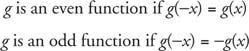for every value of x in the domain of g. Some functions are odd, some are even, and some are neither odd nor even.

If a function is even, then the graph of the function will be symmetric with the y‐axis. Alternatively, for every point on the graph, the point (− x, − y) will also be on the graph.

If a function is odd, then the graph of the function will be symmetric with the origin. Alternatively, for every point (x, y) on the graph, the point (− x, − y) will also be on the graph.

Example 3: Graph several functions and give their periods (Figure 5).Figure 5
Drawings for Example 3.

Example 4: Graph several odd functions and give their periods (Figure  6).Figure 6
Drawings for Example 4.

Example 5: Is the function f(x) = 2 x 3 + x even, odd, or neither?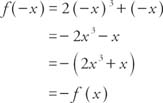Because f(−x) = − f(x), the function is odd.

Example 6: Is the function f(x) = sin x – cos x even, odd, or neither?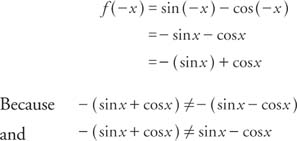the function is neither even nor odd. Note: The sum of an odd function and an even function is neither even nor odd.

Example 7: Is the function f(x) = x sin x cos x even, odd, or neither?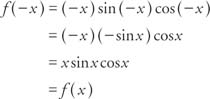Because f(− x) = f(x), the function is even.